## Equivalent Circuit of Short Transmission Line | Phasor Diagram of Short Transmission Line:

For Short Transmission Line of length 100 km or less, the total 50 Hz shunt admittance (jωCl) is small enough to be negligible resulting in the simple Equivalent Circuit of Short Transmission Line is shown in Fig. 5.1.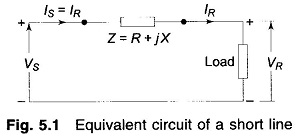This being a simple series circuit, the relationship between sending-end receiving-end voltages and currents can be immediately written as: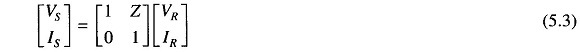The Phasor Diagram of Short Transmission Line is shown in Fig. 5.2 for the lagging current case. From this figure we can write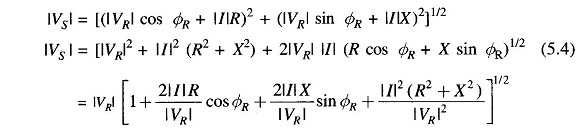The last term is usually of negligible order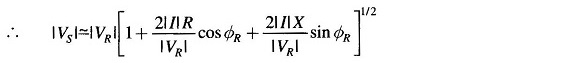Expanding binomially and retaining first order terms, we get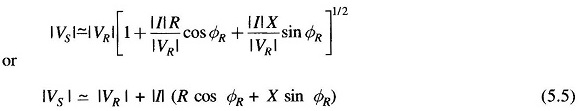The above equation is quite accurate for the normal load range.

The Fig. 5.2 shows the Phasor Diagram of Short Transmission Line for lagging current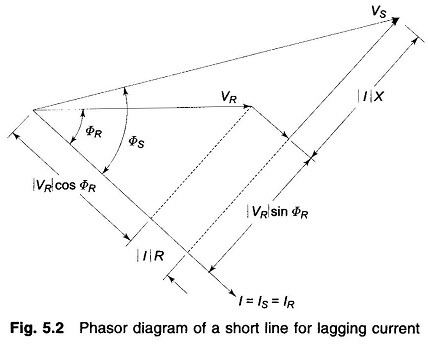Scroll to Top